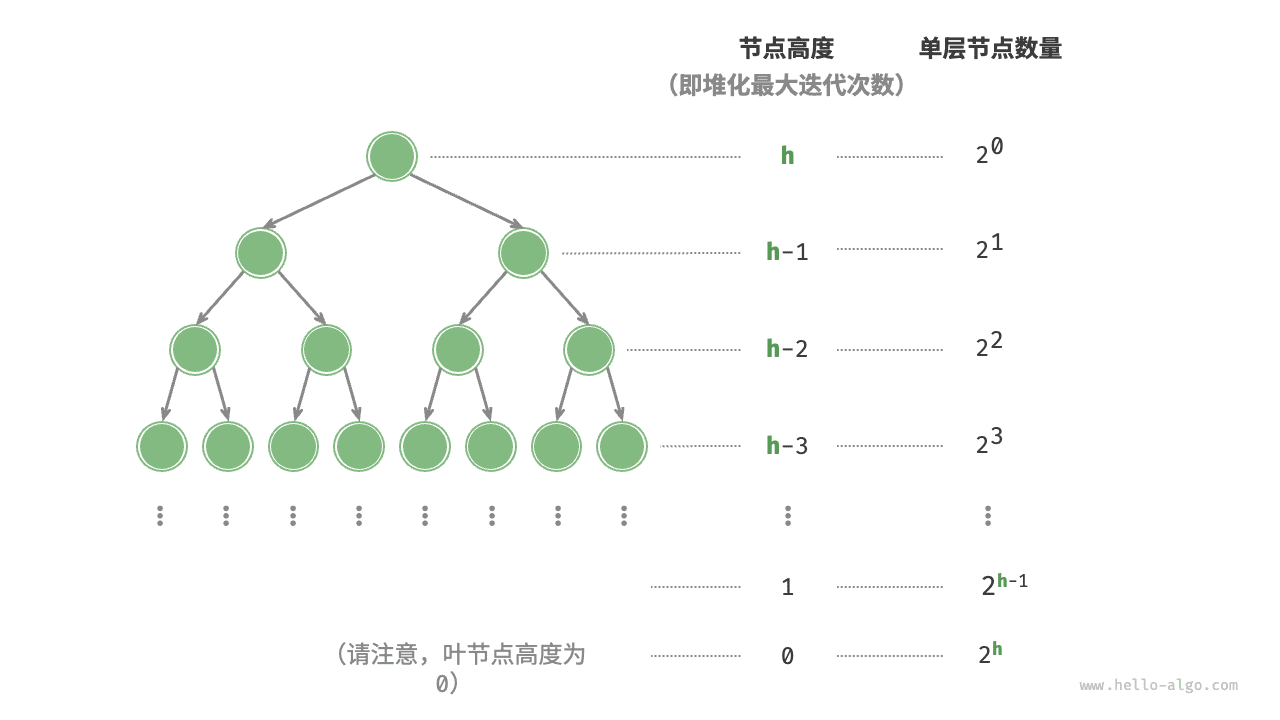# 8.2   建堆操作¶

## 8.2.2   通过遍历堆化实现¶

1. 将列表所有元素原封不动添加到堆中，此时堆的性质尚未得到满足。
2. 倒序遍历堆（即层序遍历的倒序），依次对每个非叶节点执行“从顶至底堆化”。

my_heap.py
def __init__(self, nums: list[int]):
"""构造方法，根据输入列表建堆"""
# 将列表元素原封不动添加进堆
self.max_heap = nums
# 堆化除叶节点以外的其他所有节点
for i in range(self.parent(self.size() - 1), -1, -1):
self.sift_down(i)

my_heap.cpp
/* 构造方法，根据输入列表建堆 */
MaxHeap(vector<int> nums) {
// 将列表元素原封不动添加进堆
maxHeap = nums;
// 堆化除叶节点以外的其他所有节点
for (int i = parent(size() - 1); i >= 0; i--) {
siftDown(i);
}
}

my_heap.java
/* 构造方法，根据输入列表建堆 */
MaxHeap(List<Integer> nums) {
// 将列表元素原封不动添加进堆
maxHeap = new ArrayList<>(nums);
// 堆化除叶节点以外的其他所有节点
for (int i = parent(size() - 1); i >= 0; i--) {
siftDown(i);
}
}

my_heap.cs
/* 构造函数，根据输入列表建堆 */
MaxHeap(IEnumerable<int> nums) {
// 将列表元素原封不动添加进堆
maxHeap = new List<int>(nums);
// 堆化除叶节点以外的其他所有节点
var size = parent(this.size() - 1);
for (int i = size; i >= 0; i--) {
siftDown(i);
}
}

my_heap.go
/* 构造函数，根据切片建堆 */
func newMaxHeap(nums []any) *maxHeap {
// 将列表元素原封不动添加进堆
h := &maxHeap{data: nums}
for i := h.parent(len(h.data) - 1); i >= 0; i-- {
// 堆化除叶节点以外的其他所有节点
h.siftDown(i)
}
return h
}

my_heap.swift
/* 构造方法，根据输入列表建堆 */
init(nums: [Int]) {
// 将列表元素原封不动添加进堆
maxHeap = nums
// 堆化除叶节点以外的其他所有节点
for i in stride(from: parent(i: size() - 1), through: 0, by: -1) {
siftDown(i: i)
}
}

my_heap.js
/* 构造方法，建立空堆或根据输入列表建堆 */
constructor(nums) {
// 将列表元素原封不动添加进堆
this.#maxHeap = nums === undefined ? [] : [...nums];
// 堆化除叶节点以外的其他所有节点
for (let i = this.#parent(this.size() - 1); i >= 0; i--) {
this.#siftDown(i);
}
}

my_heap.ts
/* 构造方法，建立空堆或根据输入列表建堆 */
constructor(nums?: number[]) {
// 将列表元素原封不动添加进堆
this.maxHeap = nums === undefined ? [] : [...nums];
// 堆化除叶节点以外的其他所有节点
for (let i = this.parent(this.size() - 1); i >= 0; i--) {
this.siftDown(i);
}
}

my_heap.dart
/* 构造方法，根据输入列表建堆 */
MaxHeap(List<int> nums) {
// 将列表元素原封不动添加进堆
_maxHeap = nums;
// 堆化除叶节点以外的其他所有节点
for (int i = _parent(size() - 1); i >= 0; i--) {
siftDown(i);
}
}

my_heap.rs
/* 构造方法，根据输入列表建堆 */
fn new(nums: Vec<i32>) -> Self {
// 将列表元素原封不动添加进堆
let mut heap = MaxHeap { max_heap: nums };
// 堆化除叶节点以外的其他所有节点
for i in (0..=Self::parent(heap.size() - 1)).rev() {
heap.sift_down(i);
}
heap
}

my_heap.c
/* 构造函数，根据切片建堆 */
maxHeap *newMaxHeap(int nums[], int size) {
// 所有元素入堆
maxHeap *h = (maxHeap *)malloc(sizeof(maxHeap));
h->size = size;
memcpy(h->data, nums, size * sizeof(int));
for (int i = parent(h, size - 1); i >= 0; i--) {
// 堆化除叶节点以外的其他所有节点
siftDown(h, i);
}
return h;
}

my_heap.zig
// 构造方法，根据输入列表建堆
fn init(self: *Self, allocator: std.mem.Allocator, nums: []const T) !void {
if (self.max_heap != null) return;
self.max_heap = std.ArrayList(T).init(allocator);
// 将列表元素原封不动添加进堆
try self.max_heap.?.appendSlice(nums);
// 堆化除叶节点以外的其他所有节点
var i: usize = parent(self.size() - 1) + 1;
while (i > 0) : (i -= 1) {
try self.siftDown(i - 1);
}
}


## 8.2.3   复杂度分析¶

• 假设完全二叉树的节点数量为 $$n$$ ，则叶节点数量为 $$(n + 1) / 2$$ ，其中 $$/$$ 为向下整除。因此需要堆化的节点数量为 $$(n - 1) / 2$$
• 在从顶至底堆化的过程中，每个节点最多堆化到叶节点，因此最大迭代次数为二叉树高度 $$\log n$$$T(h) = 2^0h + 2^1(h-1) + 2^2(h-2) + \dots + 2^{(h-1)}\times1$

\begin{aligned} T(h) & = 2^0h + 2^1(h-1) + 2^2(h-2) + \dots + 2^{h-1}\times1 \newline 2 T(h) & = 2^1h + 2^2(h-1) + 2^3(h-2) + \dots + 2^{h}\times1 \newline \end{aligned}

$2T(h) - T(h) = T(h) = -2^0h + 2^1 + 2^2 + \dots + 2^{h-1} + 2^h$

\begin{aligned} T(h) & = 2 \frac{1 - 2^h}{1 - 2} - h \newline & = 2^{h+1} - h - 2 \newline & = O(2^h) \end{aligned}